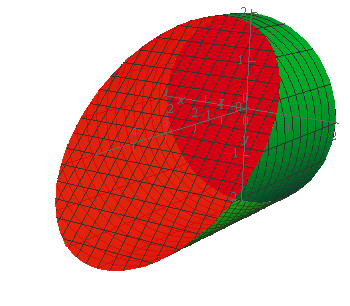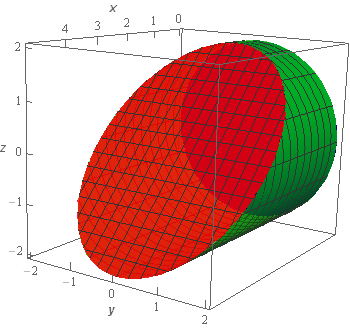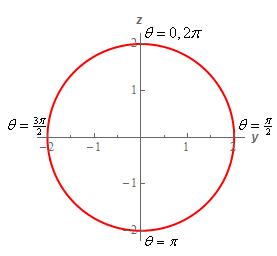Paul's Online Notes
Home / Calculus III / Surface Integrals / Surface Integrals of Vector Fields
Show Mobile Notice Show All Notes Hide All Notes
Mobile Notice
You appear to be on a device with a "narrow" screen width (i.e. you are probably on a mobile phone). Due to the nature of the mathematics on this site it is best views in landscape mode. If your device is not in landscape mode many of the equations will run off the side of your device (should be able to scroll to see them) and some of the menu items will be cut off due to the narrow screen width.

### Section 17.4 : Surface Integrals of Vector Fields

3. Evaluate $$\displaystyle \iint\limits_{S}{{\vec F\centerdot \,d\vec S}}$$ where $$\vec F = {x^2}\,\vec i + 2z\,\vec j - 3y\,\vec k$$ and $$S$$ is the portion of $${y^2} + {z^2} = 4$$ between $$x = 0$$ and $$x = 3 - z$$ oriented outwards (i.e. away from the $$x$$-axis).

Show All Steps Hide All Steps

Start Solution

Let’s start off with a quick sketch of the surface we are working with in this problem.Note that the surface in this problem is only the cylinder itself. The “caps” of the cylinder are not part of this surface despite the red “cap” in the sketch. That was included in the sketch to make the front edge of the cylinder clear in the sketch. We would only include the “caps” if the problem had specified that in some manner to make it clear.

Show Step 2

Let’s get the integral set up now. In this case the we are integrating over a cylinder and so we’ll need to set up a parameterization for the surface.

We saw how to parameterize a cylinder in the first section of this chapter so we won’t go into detail for the parameterization. The parameterization is,

$\vec r\left( {x,\theta } \right) = \left\langle {x,2\sin \theta ,2\cos \theta } \right\rangle \hspace{0.25in}\,\,\,\,0 \le \theta \le 2\pi ,\,\,\,\,\,0 \le x \le 3 - z = 3 - 2\cos \theta$

We’ll use the full range of $$\theta$$ since we are allowing it to rotate all the way around the x‑axis. The $$x$$ limits come from the two planes that “bound” the cylinder and we’ll need to convert the upper limit using the parameterization.

Next, we’ll need to compute the cross product.

${\vec r_x} = \left\langle {1,0,0} \right\rangle \hspace{0.25in}\hspace{0.25in}{\vec r_\theta } = \left\langle {0,2\cos \theta , - 2\sin \theta } \right\rangle$ ${\vec r_x} \times {\vec r_\theta } = \left| {\begin{array}{*{20}{c}}{\vec i}&{\vec j}&{\vec k}\\1&0&0\\0&{2\cos \theta }&{ - 2\sin \theta }\end{array}} \right| = 2\sin \theta \vec j + 2\cos \theta \vec k$

A unit normal vector for the surface is then,

$\vec n = \frac{{{{\vec r}_x} \times {{\vec r}_\theta }}}{{\left\| {{{\vec r}_x} \times {{\vec r}_\theta }} \right\|}} = \frac{{\left\langle {0,2\sin \theta ,2\cos \theta } \right\rangle }}{{\left\| {{{\vec r}_x} \times {{\vec r}_\theta }} \right\|}}$

We didn’t compute the magnitude of the cross product since we know that it will just cancel out when we start working with the integral.

Now we need to determine if this vector has the correct orientation. First let’s look at the cylinder from in front of the cylinder and directly along the $$x$$-axis. This is what we’d see.In this sketch the $$x$$ axis will be coming straight out of the sketch at the origin. Plugging in a few value of $$\theta$$ into the parameterization we can see that we’ll be at the points listed above.

Now, in the range $$0 \le \theta \le \frac{1}{2}\pi$$ we know that sine and cosine are both positive and so in the normal vector both the $$y$$ and $$z$$ components will be positive. This means that in the 1st quadrant above the normal vector would need to be pointing out away from the origin. This is exactly what we need to see since the orientation was given as pointing away from the $$x$$-axis and recall that the $$x$$-axis is coming straight out of the sketch from the origin.

Next, if we look at $$\frac{1}{2}\pi \le \theta \le \pi$$ (so we’re in the 4th quadrant of the graph above….) we know that in this range sine is still positive but cosine is now negative. From our unit vector above this means that the $$y$$ component is positive (so pointing in positive $$y$$ direction) and the $$z$$ component is negative (so pointing in negative $$z$$ direction). Together this again means that we have to be pointing away from the origin in the 4th quadrant which is again the orientation we want.

We could continue in this fashion looking at the remaining two quadrants but once we’ve done a couple and gotten the correct orientation we know we’ll continue to get the correct orientation for the rest.

Show Step 3

Next, we’ll need to compute the following dot product.

\begin{align*}\vec F\left( {\vec r\left( {x,\theta } \right)} \right)\centerdot \vec n & = \left\langle {{x^2},2\left( {2\cos \theta } \right), - 3\left( {2\sin \theta } \right)} \right\rangle \centerdot \frac{{\left\langle {0,2\sin \theta ,2\cos \theta } \right\rangle }}{{\left\| {{{\vec r}_x} \times {{\vec r}_\theta }} \right\|}}\\ & = \frac{1}{{\left\| {{{\vec r}_x} \times {{\vec r}_\theta }} \right\|}}\left( { - 4\sin \theta \cos \theta } \right)\end{align*}

Remember that we needed to plug in the parameterization for the surface into the vector field!

The integral is then,

\begin{align*}\iint\limits_{S}{{\vec F\centerdot \,d\vec S}} & = \iint\limits_{S}{{\frac{1}{{\left\| {{{\vec r}_x} \times {{\vec r}_\theta }} \right\|}}\left( { - 4\sin \theta \cos \theta } \right)\,dS}}\\ & = \iint\limits_{D}{{\frac{1}{{\left\| {{{\vec r}_x} \times {{\vec r}_\theta }} \right\|}}\left( { - 4\sin \theta \cos \theta } \right)\left\| {{{\vec r}_x} \times {{\vec r}_\theta }} \right\|\,dA}}\\ & = \iint\limits_{D}{{ - 4\sin \theta \cos \theta \,dA}}\end{align*}

As noted above we didn’t need to compute the magnitude of the cross product since it would just cancel out when we converted the surface integral into a “normal” double integral.

Also, recall that $$D$$ is given by the limits on $$x$$ and $$\theta$$ we found at the start of Step 2.

Show Step 4

Now all that we need to do is evaluate the double integral and that shouldn’t be too difficult at this point.

The integral is then,

\begin{align*}\iint\limits_{S}{{\vec F\centerdot \,d\vec S}} & = \iint\limits_{D}{{ - 4\sin \theta \cos \theta \,dA}}\\ & = \int_{0}^{{2\pi }}{{\int_{0}^{{3 - 2\cos \theta }}{{ - 4\sin \theta \cos \theta \,dx}}\,d\theta }}\\ & = \int_{0}^{{2\pi }}{{\left. { - 4x\sin \theta \cos \theta } \right|_0^{3 - 2\cos \theta }\,d\theta }}\\ & = \int_{0}^{{2\pi }}{{ - 4\left( {3 - 2\cos \theta } \right)\sin \theta \cos \theta \,d\theta }}\\ & = \int_{0}^{{2\pi }}{{ - 12\sin \theta \cos \theta + 8\sin \theta {{\cos }^2}\theta \,d\theta }}\\ & = \int_{0}^{{2\pi }}{{ - 6\sin \left( {2\theta } \right) + 8\sin \theta {{\cos }^2}\theta \,d\theta }}\\ & = \left. {\left( { 3\cos \left( {2\theta } \right) - \frac{8}{3}{{\cos }^3}\theta } \right)} \right|_0^{2\pi } = \require{bbox} \bbox[2pt,border:1px solid black]{0}\end{align*}